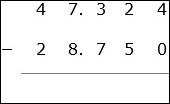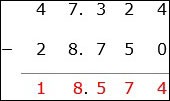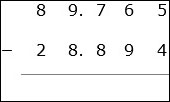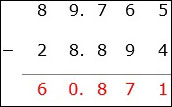Advanced subtraction of decimals is similar to subtraction of whole numbers with borrowing.

• First the decimals are aligned so that the same place values are there above and below each other.

• We then add zeros to the right of decimal numbers where ever necessary so that all numbers have the same number of digits after the decimal point.

• We begin with the rightmost column and start subtracting the digits.

• Then we keep moving to the left till all columns are subtracted.

Subtract 47.324 − 28.75

### Solution

Step 1:The decimals are aligned as shown. A zero is added to the right of 28.75 as the maximum number of decimal places is 3.

Step 2:

We start with the right most column. Subtract 4 − 0 = 4; then moving to left column, we borrow one from left; 3 becomes 2 and 12 − 5 = 7; again borrowing 1 from left; 7 becomes 6 and 12 − 7 = 5; next borrowing 1 from left; 4 becomes 3 and 16 − 8 = 8; 3 – 2 = 1

Step 3:

So the difference of the decimals is 18.574Subtract 89.765 − 28.894

### Solution

Step 1:The decimals are aligned as shown.

Step 2:

We start with the right most column. Subtract 5 − 4 = 1; then moving to left column, 6 < 9, So we borrow one from left; 7 becomes 6 and 16 − 9 = 7; 6 < 8, so borrowing 1 from left; 9 becomes 8 and 16 − 8 = 8; next 8 − 8 = 0; and 8 – 2 = 6

Step 3:

So the difference of the decimals is 60.871